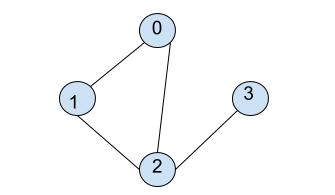New update is available. Click here to update.

# BFS in Graph

Last Updated: 7 Dec, 2020
Difficulty: Easy

## PROBLEM STATEMENT

#### Note:

``````1. Here, you need to consider that you need to print the BFS path starting from vertex 0 only.
2. V is the number of vertices present in graph G, and all vertices are numbered from 0 to V-1.
3. E is the number of edges present in graph G.
4. Graph input is provided as the number of vertices and a list of edges.
5. Handle for Disconnected Graphs as well.
``````

#### For Example: Consider graph:``````Here, starting from 0, it is connected to 1 and 2 so, BFS traversal from here will be [0, 1, 2 ]. Now, 3 is also connected to 2. So, BFS traversal becomes [0, 1, 2, 3].
``````

#### Note:

``````For each node, the correct order of printing the connected nodes will be sorted order, i.e., if {3,6,9,4} are connected to 1, then the correct order of their printing is {1,3,4,6,9}.
``````

#### Input Format :

``````The first line of input contains two integers that denote the value of V and E.
Each of the following E lines contains space-separated two integers that denote an edge between vertex A and B.
``````

#### Output Format :

``````For each test case, print the BFS Traversal, as described in the task.
Output for each test case will be printed in a separate line.
``````

#### Note:

``````You do not need to print anything; it has already been taken care of. Just implement the given function.
``````

#### Constraints :

``````0 <= V <= 10^4
0 <= E <= (V * (V - 1)) / 2
0 <= A <= V - 1
0 <= B <= V - 1

Where 'V' is the number of vertices, 'E' is the number of edges, 'A' and 'B' are the vertex numbers.
Time Limit: 1 second
``````## Approach 1

Let us consider a function BFS that accepts a list of edges, EDGES, and the number of vertices VERTEX as a parameter and do:

2. Define a boolean array VISITED to store nodes that are already visited.
3. Iterate over VISITED[i] for each 0<= i < VISITED.LENGTH, and check:
1. If VISITED[i] is FALSE, call BFSHELPER function for ADJACENCYMATRIX, i and VISITED.

Now, the function BFSHELPER accepts as parameters, an adjacency matrix of edges ADJACENCYMATRIX, an integer SOURCE and boolean array VISITED and do:

1. Define a queue, ADJNODE, to store nodes under consideration but not added to the result.
2. Create an ArrayList RESULT.
3. Mark VISITED[SOURCE] as TRUE.